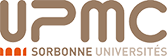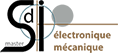Internship validating the first year of acoustics master at UPMC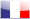Version française

Caracterisation of damping and associated impact noise measuring in viscoelastic materials.
• Context
• Damping Estimation
• Vibro-Acoustic Measurements
• Synthesis

### Context

 This internship is part of the research of Benjamin COTTE and Augustin PARRET-FREAUD in time domain simulation of the vibro-acoustic behavior of a structure subjected to an impact. To model the vibration of the structure different mechanical properties of materials that constitutes it must be know. The damping is fundamental, it defines how the vibrations will be amortized in each material as a function of frequency. Their current work therefore focuses especially on the developement of temporal damping models to represent the behavior of a wide range of viscoelastic materials (wood, polymers, composites, ...). Their approach is to model this setting as a time digital filter. Our work is thus part of this project with the goal to measure the viscoelastic damping in different materials. The obtained values ​​will be use as database for futur works. A second part of the internship is to measure the impact of noise of different materials plates and compare our results with those of a simple model of radiation.

### Damping Estimation

#### Estimation method

We excite plates of different materials with a swept sine frequency. These plates are free on their edges and excited at their centers by a shaker, as shown in the figure below. Signals from the force sensor and accelerometer is acquired. The accelerometer signal is then convolved with the inverse flitre. We then obtain the impulse response (IR) of the plate on frequency range excited.

Once the IR calculated, we realize a STFT thanks to the sepctrogram Matlab function. On each frequency band we calculate an energie decay curve. Then a linear regression is made ​​over the beginning of the curve and the damping coefficent is obtained thanks to the calculated slope. You can see an example of spectrogram in the figure below. It can be seen that the damping increases with frequency because the vibration decay is faster.

#### Improved method

Our work, as a first step, carried on the improvement of this method. A study of the entire spectrum had not yet be completed and the first study seemed to show a slight dependence of the result at the time of measurement. In addition, parasites on the limits of study band was very active in previous studies, which make impossible the measurement at these frequencies.

Then, convergence tests were realised to know the correct value of the acquisition time to use. For a global and applicable to all studies criterion we introduce the scanning speed (number of excited Hertz per second), which varies depending on the frequency because we do not take a linear swept sine. After such a study on our plates, we then concluded that we need a scan passing at least 0.6 s by mode.

It then seeks to ensure the lowest possible modal recovery. Indeed, it is assumed that the scanning speed can change the apparent "width" of mode and therefore the associated damping. We then established another criterion: the ratio between the characteristic decay time of a mode and modal spacing. After several tests, we conclude that this report must be at least 10, which has a clear physical meaning.

It is then the noise suppression in band limits that interested us. Indeed, they prevent us from estimating damping on those parts of the spectrum. We then propose apodize the excitation signal. So, we create a window so that the unitary part of the window is at the frequency band to study i.e. the excitement is higher bandwidth than necessary.

Other criteria, this time on the analysis, so the estimate, strictly speaking, have been a reflection. We then defined frequency bands in which the temporal and frequency resolution is fixed criterion functions such as time decay mode or the presence of at least one mode in a frequency band $\Delta f$.

#### Results

With all these criteria then we can estimate the damping over the entire audible spectrum and for different materials. Here, the estimation result of the damping in the nylon.

 This experiment aims to observe the influence of damping on the impact noise. For this we compare the results of a relatively simple model to the measurements obtained. Later, these measures will also allow to validate Augustin Parret-Fréaud's temporal simulations. So we want to measure the impact force and displacement for modeling the impact. We also want to measure the vibration of the plate for comparison with our model. Finally, we want to measure the acoustic pressure field. The experiment is carried out in the anechoic chamber ENSTA. It has an impactor, two accelerometers and two microphones, as shown in the following figures.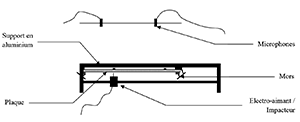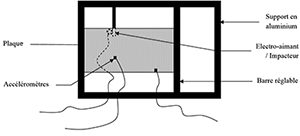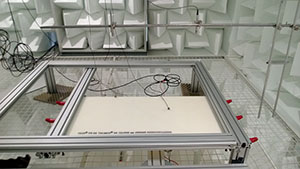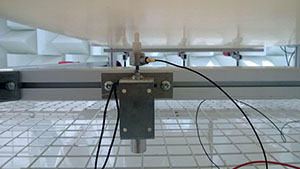We were then able, through a force sensor, to measure the force of impact. Knowing the position of this excitation, it is then possible to determine the operational shape of the plate. We have, for each acquisition, the recorded acceleration signals and pressures. For the PA66 Nylon 13mm thick plate, it is proposed to compare the spectra of two accelerometers with mode shapes calculated, see figure below. We can see that at this frequency, we have amplitude differences between the accelerations that fit well with mode shape annaliticaly calculated.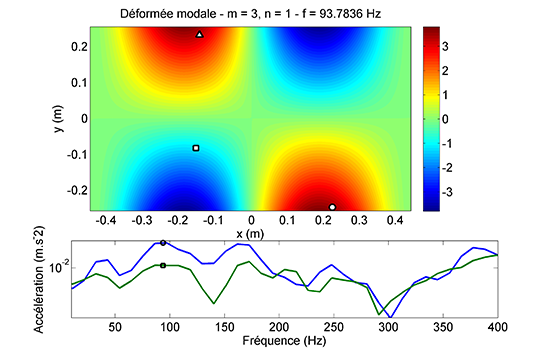Mode shape with the placement of the impactor (triangle) and the two accelerometers (square and circle) and their associated spectra. We now turn to modeling the radiated acoustic field thanks to the Rayleigh integral calculated for only two points corresponding to the positions of the two microphones wich location have been measured. The model results are compared with measurements in an anechoic chamber in the following figure. We can see that the magnitudes are good.Comparison model/experience for the micro 1 (left) and micro 2 (right)